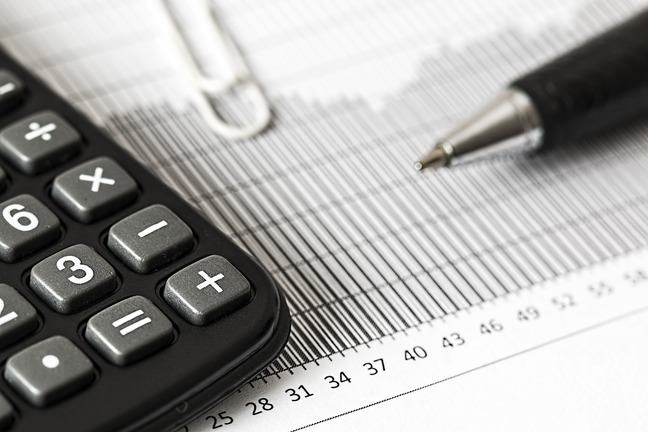## Want to keep learning?

This content is taken from the SOAS University of London's online course, Risk Management in the Global Economy. Join the course to learn more.
2.8

## SOAS University of London# Return and risk of financial securities

Before we consider the risk of a portfolio of assets, we have to look at the risk of the individual securities which are included in the portfolio. This activity will require some calculations. The calculations are very straightforward, as they just require some simple additions and multiplications, and can be easily carried out with a standard calculator or on a spreadsheet.

The risk of a security is related to the variability of its returns. Suppose we have an asset $$X$$, which could be a bond or a stock. For simplicity, its return $$R_X$$ can be equal to 10%, 6% or 2% with probability 20%, 60% and 20% (or 1/5, 3/5 and 1/5) respectively. We can summarise the return on $$X$$ with the following table.

Probability Return: $$R_X$$
0.20 10$$\%$$
0.60 6$$\%$$
0.20 2$$\%$$

How can we measure the variability of the returns on $$X$$?

First, let’s compute the expected return on $$X$$, or the mean return on $$X$$. This is indicated by $$\mu_X$$, or sometimes $$E(R_X)$$. It is a measure of the average return, and can be calculated as each possible return multiplied by the probability it will occur:

$\mu_X = (0.20 \times 0.10) + (0.60 \times 0.06) + (0.20 \times 0.02) = 0.06 = 6\%$

The expected return on asset $$X$$ is 6%. This is a measure of what investors can expect to gain by investing in the asset. However, in reality the return can be as high as 10% or as low as 2%. We cannot be certain of our return: the return on asset $$X$$ is risky. Clearly, the riskiness of $$X$$ is due to the fact that the actual return can be higher, or lower, than the expected return. The risk of the asset is therefore due to its variability.

How can we measure the variability of the returns on the asset?

To do this, we compute the variance of the returns. This is a measure of how much the actual returns can vary around the mean. In order to compute the variance, we start by taking the difference of each possible return from the mean. We have already seen the mean is equal to 6%:

Probability Return: difference from the mean $$R_X - \mu_X$$
0.20 10$$\%$$ – 6$$\%$$ = 4$$\%$$ = 0.04
0.60 6$$\%$$ – 6$$\%$$ = 0$$\%$$ = 0.00
0.20 2$$\%$$ – 6$$\%$$ = –4$$\%$$ = –0.04

The difference between each return and the mean can be positive or negative, depending on whether the return is higher or lower than the mean return of 6%. We thus square the differences of the returns from the mean (can you see why we need to do this step?):

Probability Return: difference from the mean, squared $$(R_X - {{\mu_X}^2})$$
0.20 0.0016
0.60 0
0.20 0.0016

Finally, we take an average of the squared differences from the mean, again using the probability that each return will occur. This is the variance of the returns, $$\sigma_x^2$$ :

$\sigma_x^2 = (0.20 \times 0.0016) + (0.60 \times 0) + (0.20 \times 0.0016) = 0.00064$

The variance is a measure of the variability of the returns. The higher the variance, the greater the dispersion of the returns and therefore the riskier the security.

An easier measure to interpret is the standard deviation, which is computed as the square root of the variance and is denoted as $$\sigma_x$$:

$\sigma_x = \sqrt {{\sigma}^2_x}$

In our example, the standard deviation is:

$\sigma_x = \sqrt 0.00064 = 0.0253 = 2.53\%$

How do we interpret the standard deviation of the returns? The larger the standard deviation, the greater the uncertainty associated with the returns on the asset. The standard deviation can therefore be regarded as a measure of the risk of the asset.

To see how the standard deviation is related to the variability of the returns on the asset, let us consider a second security $$Y$$ whose possible returns $$R_Y$$ are shown in the following table:

Probability Return: $$R_Y$$
0.20 16%
0.60 6%
0.20 -4%

Compare the returns on $$X$$ with the returns on $$Y$$. You will see that when things are good for asset $$Y$$, they are very good (16%), but when they are bad, they are very bad indeed (–4%) and we make a loss. The expected return on $$Y$$, $$\mu_Y$$, or $$E(R_Y)$$, is computed as:

$\mu_Y = (0.20 \times 0.16) + (0.60 \times 0.06) + (0.20 \times (-0.04))= 0.06 = 6\%$

The expected return on asset $$Y$$ is 6%, the same as for asset $$X$$. On average, the two securities are expected to have the same return. However, asset $$Y$$ looks riskier than asset $$X$$: asset $$Y$$ could generate larger profits but it could also generate losses. What will the standard deviation for $$Y$$ look like? Let us first compute the variance. We can use the following table:

Probability $$R_Y - \mu_Y$$ $$(R_Y - \mu_Y)^2$$
0.20 16% – 6% = 10% = 0.10 0.01
0.60 6% – 6% = 0% = 0.00 0
0.20 –4% – 6% = –10% = –0.10 0.01

The variance $$\sigma_Y^2$$ is:

$\sigma_Y^2 = (0.20 \times 0.01) + (0.60 \times 0) + (0.20 \times 0.01) = 0.004$

and the standard deviation $$\sigma_Y$$ is:

$\sigma_Y = \sqrt {{\sigma}^2_Y} = \sqrt 0.004 = 0.0632 = 6.32\%$

The standard deviation of $$Y$$, $$\sigma_Y = 6.32\%$$, is larger than the standard deviation of $$X$$, $$\sigma_X =2.53\%$$. Asset $$Y$$ has a greater variability than asset $$X$$.

Can you see how the standard deviation of the returns is a measure of the risk of the asset?